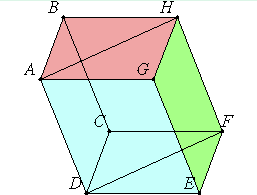# Definition 24

If a solid is contained by parallel planes, then the opposite planes in it are equal and parallelogrammic.

Let the solid CDHG be contained by the parallel planes AC, GF, AH, DF, BF, and AE.

I say that the opposite planes in it are equal and parallelogrammic.XI.16

Since the two parallel planes BG and CE are cut by the plane AC, therefore their common sections are parallel. Therefore AB is parallel to DC. Again, since the two parallel planes BF and AE are cut by the plane AC, therefore their intersections are parallel. Therefore BC is parallel to AD.

But AB was proved parallel to DC, therefore AC is a parallelogram. Similarly we can prove that each of the planes DF, FG, GB, BF, and AE is a parallelogram.

Join AH and DF.

XI.10

Then, since AB is parallel to DC, and BH is parallel to CF, therefore the two straight lines AB and BH, which meet one another, are parallel to the two straight lines DC and CF, which meet one another, not in the same plane. Therefore they contain equal angles. Therefore the angle ABH equals the angle DCF.

And, since the two sides AB and BH equal the two sides DC and CF, and the angle ABH equals the angle DCF, therefore the base AH equals the base DF, and the triangle ABH equals the triangle DCF.

I.34

And the parallelogram BG is double the triangle ABH, and the parallelogram CE is double the triangle DCF, therefore the parallelogram BG equals the parallelogram CE.

Similarly we can prove that AC equals GF, and AE equals BF.

Therefore, if a solid is contained by parallel planes, then the opposite planes in it are equal and parallelogrammic.

Q.E.D.

## Guide

The statement of the theorem is not sufficiently detailed. All three of the octahedron, icosahedron, and dodecahedron (see XI.Def.26-28) are contained by parallel planes, but their faces are triangles or pentagons, not parallelograms. They do not have six faces, however, but eight, twenty, or twelve.

The correct hypothesis for this proposition is that the solid is contained by three pairs of parallel planes. Then the intersection of each plane with the other four nonparallel planes can be shown to be sides of a parallelgram, and the parallelograms on opposite planes can be shown to be congruent, what Euclid would call similar and equal parallelograms. That the opposite parallelograms are not just equal but also similar should be stated in the conclusion of the proposition.

#### Parallelepipeds

The term “parallelepipedal solid,” abbreviated as “parallelepiped,” is used for the solid treated by this proposition. It can be defined as a solid bounded by three pairs of parallel faces. Then this proposition shows that a parallelepiped has the further properties that each face is a parallelogram, and opposite parallelograms have parallel and equal corresponding sides, and equal corresponding angles.

Parallelepipeds are to solid geometry what parallelograms are to plane geometry. This proposition is the analogue of proposition I.34 which introduces parallelograms just as this proposition introduces parallelepipeds. It is likely that both are the product of Euclid’s own research.

#### Use of this proposition

This proposition is used in the next as well as others in this book and the next.